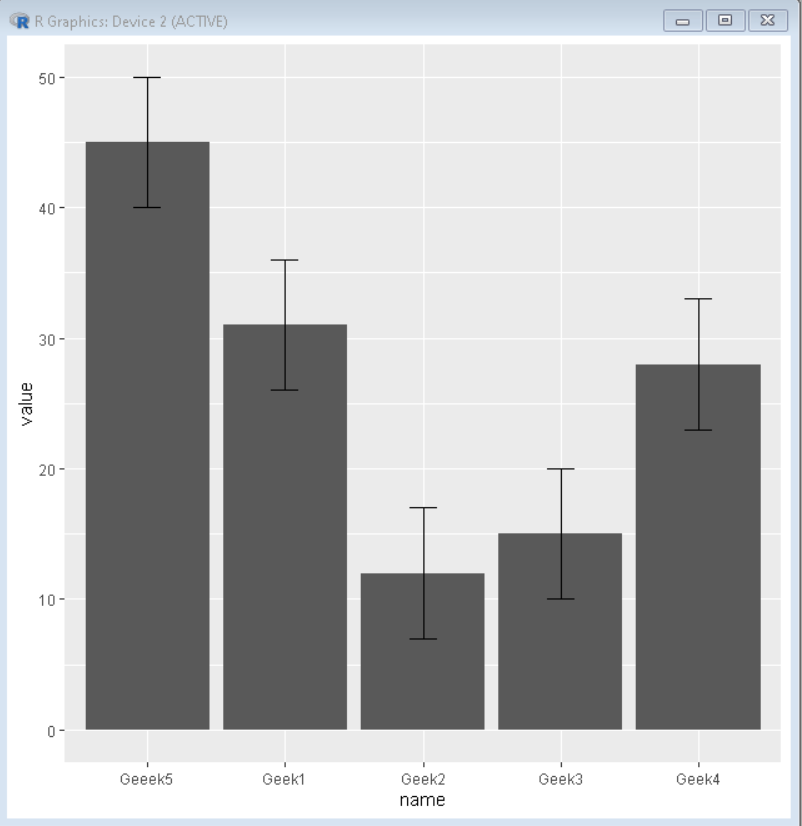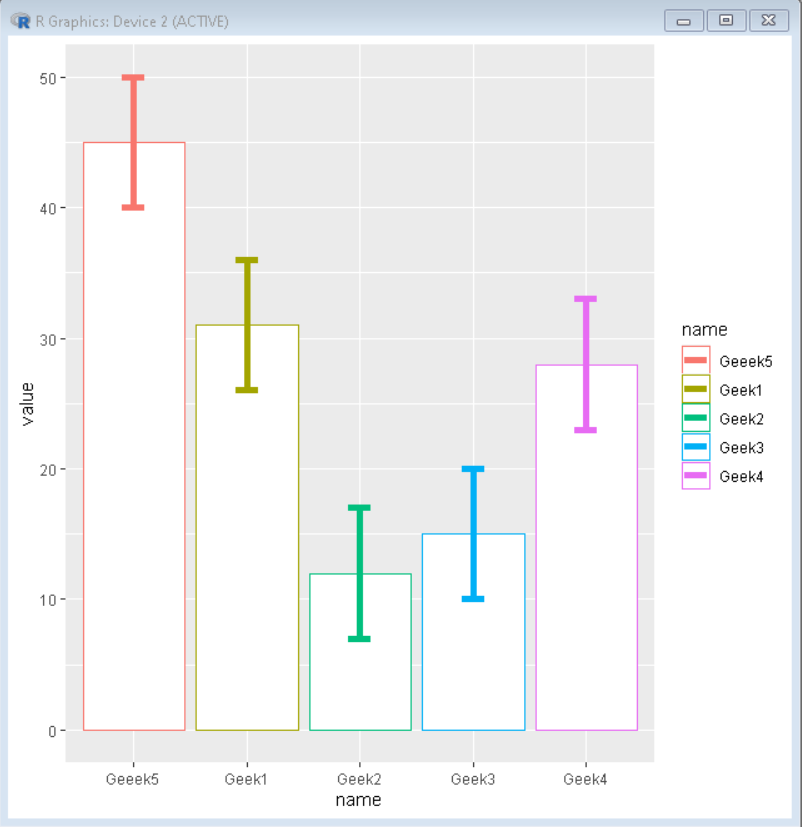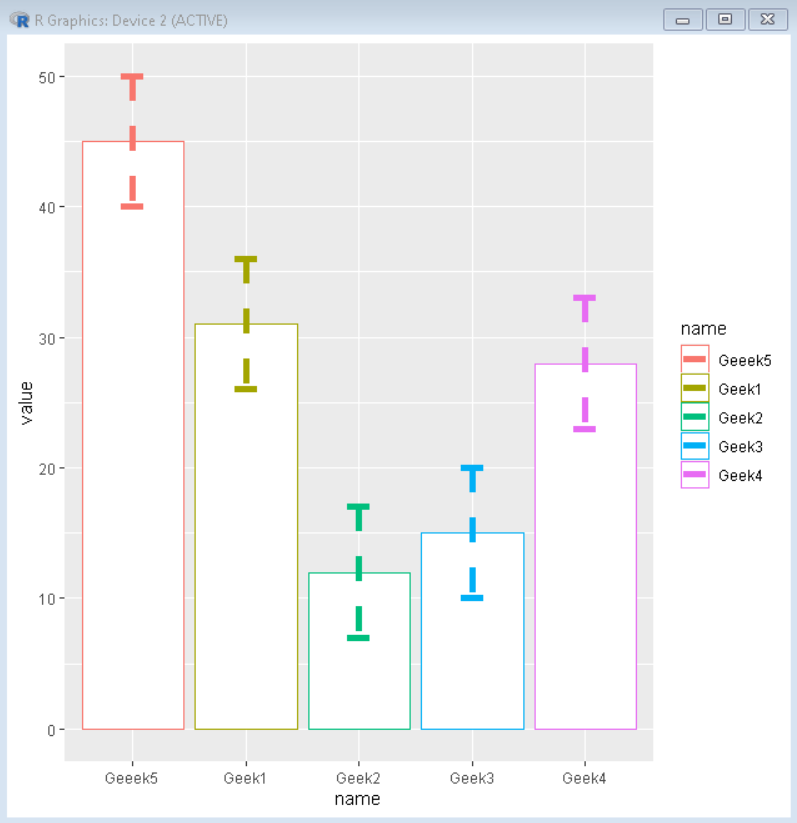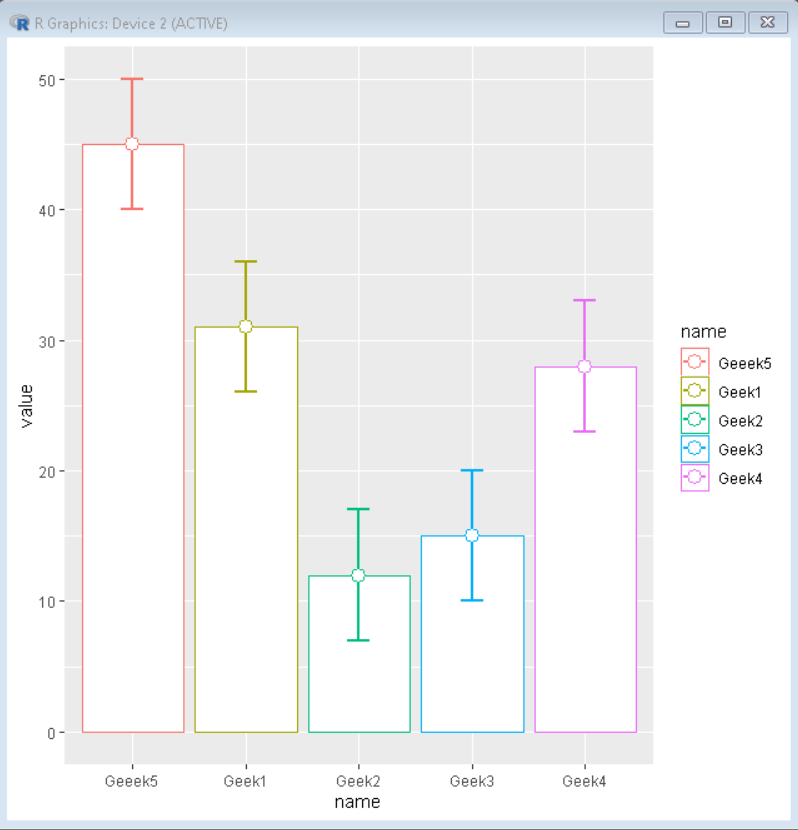# How To Make Barplots with Error bars in ggplot2 in R?

• Last Updated : 12 Nov, 2021

In this article, we will discuss how to make a barplot with an error bar using ggplot2 in the R programming language.

Error Bars helps us to visualize the distribution of the data. Error Bars can be applied to any type of plot, to provide an additional layer of detail on the presented data. Often there may be uncertainty around the count values in data due to error margins and we could represent them as an error bar. Error bars are used to show the range of uncertainty around the distribution of data.

We can draw error bars to a plot using the geom_errorbar() function of the ggplot2 package of the R Language.

Syntax: plot + geom_errorbar( aes( ymin= value – standard_error, ymax= value + standard_error ))

where,

value: determines the column for mean values

standard_error: determines the mean error

## Basic Barplots with Error bars in ggplot2

Here is a basic bar plot with error bars plotted on top using the geom_errorbar() function.

## R

 `# Create sample data` `set.seed``(5642)                             ` `sample_data <- ``data.frame``(name = ``c``(``"Geek1"``,``"Geek2"``,` `                                   ``"Geek3"``,``"Geek4"``,` `                                   ``"Geeek5"``) ,` `                          ``value = ``c``(31,12,15,28,45)) `   `# create standard error` `standard_error = 5`   `# Load ggplot2 package` `library``(``"ggplot2"``) `   `# Create bar plot using ggplot() function` `ggplot``(sample_data,` `             ``aes``(name,value)) + `   `# geom_bar function is used to plot bars of barplot` `geom_bar``(stat = ``"identity"``)+`   `# geom_errorbar function is used to plot error bars` `geom_errorbar``(``aes``(ymin=value-standard_error, ` `                    ``ymax=value+standard_error),` `                ``width=.2)`

Output:## Color customization

We can color customize the error bars to suit our requirements using the color, width, and size parameter of the geom_errorbar() function.

Syntax: plot + geom_errorbar( aes( ymin, ymax, color ), width, size)

where,

• color: determines the color of the error bar
• width: determines the horizontal spread of the error bar.
• size: determines the thickness of the error bar.

Here, is a barplot with error bars colored by column name. Error bars are formatted using width and size parameters to give contrasting appeal and look.

## R

 `# Create sample data` `set.seed``(5642)                             ` `sample_data <- ``data.frame``(name = ``c``(``"Geek1"``,``"Geek2"``,` `                                   ``"Geek3"``,``"Geek4"``,` `                                   ``"Geeek5"``) ,` `                          ``value = ``c``(31,12,15,28,45)) `   `# create standard error` `standard_error = 5`   `# Load ggplot2 package` `library``(``"ggplot2"``) `   `# Create bar plot using ggplot() function` `ggplot``(sample_data,` `             ``aes``(name,value,, color=name)) + `   `# geom_bar function is used to plot bars of barplot` `geom_bar``(stat = ``"identity"``, fill=``"white"``)+`   `# geom_errorbar function is used to plot error bars` `# color width and size parameter are used to format` `# the error bars` `geom_errorbar``(``aes``(ymin=value-standard_error, ` `                    ``ymax=value+standard_error, color=name),` `                ``width=0.2, size=2)`

Output:## Line type customization

We can customize the line shape of the error bar using the linetype parameter of the geom_errorbar function of the ggplot2 package in the R Language.

Syntax: plot + geom_errorbar( aes( ymin, ymax ), linetype)

where,

• linetype: determines the shape of stroke of the error bar

Here, is a basic ggplot2 bar plot with error bars with striped lines made using the linetype property of geom_errorbar() function.

## R

 `# Create sample data` `set.seed``(5642)                             ` `sample_data <- ``data.frame``(name = ``c``(``"Geek1"``,``"Geek2"``,` `                                   ``"Geek3"``,``"Geek4"``,` `                                   ``"Geeek5"``) ,` `                          ``value = ``c``(31,12,15,28,45)) `   `# create standard error` `standard_error = 5`   `# Load ggplot2 package` `library``(``"ggplot2"``) `   `# Create bar plot using ggplot() function` `ggplot``(sample_data,` `             ``aes``(name,value,, color=name)) + `   `# geom_bar function is used to plot bars of barplot` `geom_bar``(stat = ``"identity"``, fill=``"white"``)+`   `# geom_errorbar function is used to plot error bars` `# color width and size parameter are used to ` `# format the error bars` `geom_errorbar``(``aes``(ymin=value-standard_error, ` `                    ``ymax=value+standard_error, color=name),` `                ``width=0.2, size=2, linetype=2)`

Output:## Mean point in error bar

To emphasize the mean of the error bar we can even add a point at the center of the error bar using the geom_point() function of the ggplot2 package.

Syntax: plot+ geom_point( aes( x, y), size, shape, fill)

where,

• size: determines the size of the point
• shape: determines the shape of the point
• fill: determines the fill color of the point

Here, is a bar plot with error bars and a point at the middle of the error bar made using the geom_errorbar() and the geom_point() function of the ggplot2 package.

## R

 `# Create sample data` `set.seed``(5642)                             ` `sample_data <- ``data.frame``(name = ``c``(``"Geek1"``,``"Geek2"``,` `                                   ``"Geek3"``,``"Geek4"``,` `                                   ``"Geeek5"``) ,` `                          ``value = ``c``(31,12,15,28,45)) `   `# create standard error` `standard_error = 5`   `# Load ggplot2 package` `library``(``"ggplot2"``) `   `# Create bar plot using ggplot() function` `ggplot``(sample_data,` `             ``aes``(name,value,, color=name)) + `   `# geom_bar function is used to plot bars of barplot` `geom_bar``(stat = ``"identity"``, fill=``"white"``)+`   `# geom_errorbar function is used to plot error bars` `# color width and size parameter are used to format` `# the error bars` `geom_errorbar``(``aes``(ymin=value-standard_error, ` `                    ``ymax=value+standard_error, color=name),` `                ``width=0.2, size=1, linetype=1)+`   `# geom_point is used to give a point at mean` `geom_point``(mapping=``aes``(name, value), size=4, shape=21, fill=``"white"``)`

Output:My Personal Notes arrow_drop_up
Recommended Articles
Page :